# The distribution of Fourier coefficients of half-integral weight forms

Let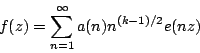be a newform of weight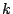for the full modular group, and let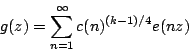be a cusp form of weight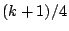and level 4 which is associated to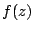by the Shimura map. We normalize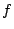by requiring that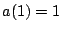and we normalize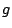by requiring that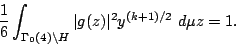Then for squarefree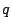with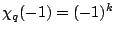we have, by the formula of Kohnen and Zagier,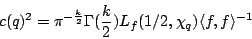where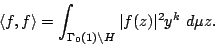In particular, the Riemann Hypothesis for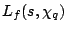implies that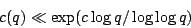for an appropriate choice of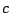. If the bound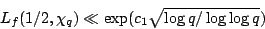holds, then a similar bound for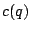will hold (but with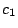replaced by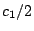). The question here is to decide which (if either) of these bounds represents the true state of affairs.

Ifhas a square factor, then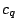can be determined from values of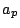where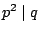. It is known, by Deligne's theorem, that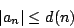where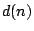is the number of divisors of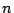. Of course,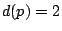so that we can write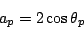where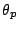is real. The conjecture of Sato and Tate about the distribution of theasserts that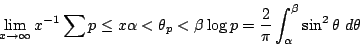for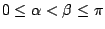. A consequence of the Sato-Tate conjecture is that there are infinitely many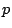(actually a positive proportion of) for which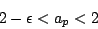for any given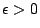. The maximum order ofis given by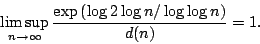The Sato-Tate conjecture implies the same assertion withreplaced by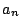.

Thus, in particular, we find that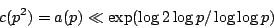and we expect this bound to be sharp in the sense that the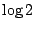cannot be replaced by anything smaller.

Thus, if the smaller'' bound is true for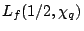then there is a great variation between the distribution of the Fourier coefficients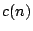according to whetheris square or squarefree.

Back to the main index for L-functions and Random Matrix Theory.